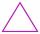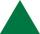3-bracket 3

Two angles in a triangle are 90° and 60°. Has triangle at least two equal sides?

Result

Solution:Leave us a comment of example and its solution (i.e. if it is still somewhat unclear...):Be the first to comment!Next similar examples:

1. 3-bracket 2May be the smallest angle in the triangle greater than 40°?
2. 3-bracketMay be the largest angle in the triangle less than 20°?
3. Triangle P2Can triangle have two right angles?
4. QuizQAn isosceles triangle has two sides of length 7 km and 39 km. How long is a third side?
5. MidpointsTriangle whose sides are midpoints of sides of triangle ABC has a perimeter 45. How long is perimeter of triangle ABC?
6. Negative in equation2x + 3 + 7x = – 24, what is the value of x?
7. NormThree workers planted 3555 seedlings of tomatoes in one dey. First worked at the standard norm, the second planted 120 seedlings more and the third 135 seedlings more than the first worker. How many seedlings were standard norm?
8. HotelThe hotel has a p floors each floor has i rooms from which the third are single and the others are double. Represents the number of beds in hotel.
9. Dropped sheetsThree consecutive sheets dropped from the book. The sum of the numbers on the pages of the dropped sheets is 273. What number has the last page of the dropped sheets?
10. Equation 29Solve next equation: 2 ( 2x + 3 ) = 8 ( 1 - x) -5 ( x -2 )
11. AlleyAlley measured a meters. At the beginning and end are planted poplar. How many we must plant poplars to get the distance between the poplars 15 meters?
12. WithdrawalIf I withdrew 2/5 of my total savings and spent 7/10 of that amount. What fraction do I have in left in my savings?
13. RoundingThe following numbers round to the thousandth:
14. Pizza 4Marcus ate half pizza on monday night. He than ate one third of the remaining pizza on Tuesday. Which of the following expressions show how much pizza marcus ate in total?
15. DecideThe rectangle is divided into seven fields. On each box is to write just one of the numbers 1, 2 and 3. Mirek argue that it can be done so that the sum of the two numbers written next to each other was always different. Zuzana (Susan) instead argue that.
16. Round it0.728 round to units, tenths, hundredths.
17. Cupcakes 2Susi has 25 cupcakes. She gives 4/5. How much does she have left?